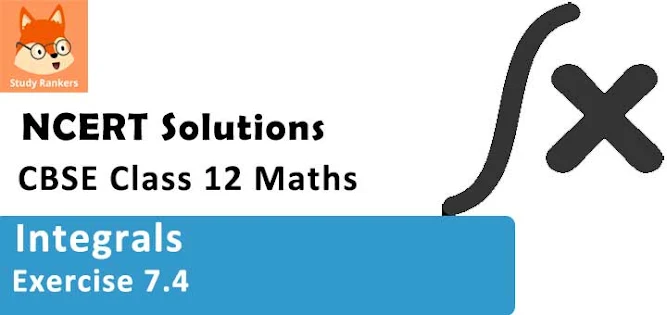# Class 12 Maths NCERT Solutions for Chapter 7 Integrals Exercise 7.4### Integrals Exercise 7.4 Solutions

1. Integrate the functions 3x2 /(x6 + 1)

Solution

2. Integrate the functions 1/√(1 + 4x2 )

Solution

3. Integrate the functions 1/√[(2 - x)2 + 1]

Solution

4. Integrate the functions 1/√(9 - 25x2 )

Solution

5. Integrate the functions 3x/(1 + 2x4 )

Solution

6. Integrate the functions x2 /(1 - x6 )

Solution

7. Integrate the functions (x - 1)/√(x2 - 1)

Solution

8. Integrate the functions x2 /√(x6 + a6 )

Solution

9. Integrate the functions sec2 x/√(tan2 x + 4)

Solution

10. Integrate the functions 1/√(x2 + 2x +2)

Solution

11. Integrate the function 1/√(9x2 + 6x + 5)

Solution

12. Integrate the functions 1/√(7 - 6x - x2 )

Solution

7 - 6x - x2 can be written as  7 - (x2 + 6x + 9 - 9).
Therefore,
7 - (x2 + 6x + 9 - 9)
= 16 - (x2 + 6x + 9)
= 16 - (x + 3)2
= (4)2 - (x + 3)2

13. Integrate the functions 1/√(x - 1)(x - 2)

Solution

14. Integrate the functions  1/√(8 + 3x - x2 )

Solution

8 + 3x - x2 can be written as 8 - (x2 - 3x + 9/4 - 9/4).
Therefore,

15. Integrate the functions 1/√[(x- a)(x - b)]

Solution

(x - a)(x - b) can be written as x2 - (a + b)x + ab.
Therefore,
x2 - (a + b)x + ab

16. Integrate the functions (4x + 1)/√(2x2 + x - 3)

Solution

17. Integrate the functions (x + 2)/√(x2 - 1)

Solution

18. Integrate the functions (5x - 2)/(1 + 2x + 3x2 )

Solution

19. Integrate the functions (6x + 7)/√[(x - 5)(x - 4)] .

Solution

x2 - 9x + 20 can be written as x2 - 9x + 20 + 81/4 - 81/4 .
Therefore,
x2 - 9x + 20 + 81/4 - 81/4

20.  Integrate the functions (x + 2)/√(4x - x2 )

Solution

21. Integrate the functions (x + 2)/√(x2 + 2x + 3)

Solution

22. Integrate the functions (x + 3)/(x2 - 2x - 5)

Solution

Let x2 - 2x - 5 = t
⇒ (2x -2)dx = dt
⇒ I1 = ∫(dt/t) = log|t| = log|x2 - 2x - 5|   .....(2)

23. Integrate the functions (5x + 3)/√(x2 + 4x + 10)

Solution
2A = 5 ⇒ A = 5/2
4A + B = 3 ⇒ B = -7

24. Choose the correct answer int. dx/(x2 + 2x + 2) equals
A. x tan−1 (x + 1) + C
B. tan− 1 (x + 1) + C
C. (x + 1) tan−1 x + C
D. tan−1 x + C

Solution

25. Choose the correct answer ∫dx/√(9x - 4x2 ) equals
(A) (1/9)sin-1 [(9x - 8)/8] + C
(B) (1/2)sin-1 [(8x - 9)/9] + C
(C) (1/3)sin-1 [(9x - 8)/8] + C
(D) (1/2)sin-1 [(9x - 8)/9] + C

Solution